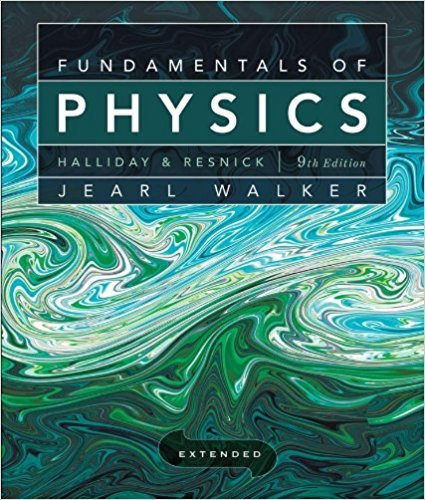×
×

# Solved: Consider the slab shown in Fig. lS-lS. SupposeISBN: 9780470469088 189

## Solution for problem 53 Chapter 18

Fundamentals of Physics Extended | 9th Edition

• Textbook Solutions
• 2901 Step-by-step solutions solved by professors and subject experts
• Get 24/7 help from StudySoup virtual teaching assistantsFundamentals of Physics Extended | 9th Edition

4 5 1 251 Reviews
19
1
Problem 53

Consider the slab shown in Fig. lS-lS. Suppose that L = 25.0 cm, A = 90.0 cm2, and the material is copper. If TH = 125C, Tc = 10.0C, and a steady state is reached, find the conduction rate through the slab.

Step-by-Step Solution:
Step 1 of 3

Chapter 16: Temperature and Heat  Heat: The energy transferred between objects because of a temperature difference o When the transfer of heat between objects in thermal contact ceases, they are in thermal equilibrium  Zeroth law of thermodynamics: if object A is in thermal equilibrium with object B, and object C is also in thermal equilibrium with object...

Step 2 of 3

Step 3 of 3

##### ISBN: 9780470469088

The answer to “Consider the slab shown in Fig. lS-lS. Suppose that L = 25.0 cm, A = 90.0 cm2, and the material is copper. If TH = 125C, Tc = 10.0C, and a steady state is reached, find the conduction rate through the slab.” is broken down into a number of easy to follow steps, and 42 words. This full solution covers the following key subjects: . This expansive textbook survival guide covers 37 chapters, and 3413 solutions. Since the solution to 53 from 18 chapter was answered, more than 236 students have viewed the full step-by-step answer. Fundamentals of Physics Extended was written by and is associated to the ISBN: 9780470469088. This textbook survival guide was created for the textbook: Fundamentals of Physics Extended, edition: 9. The full step-by-step solution to problem: 53 from chapter: 18 was answered by , our top Physics solution expert on 12/27/17, 08:15PM.

Unlock Textbook Solution